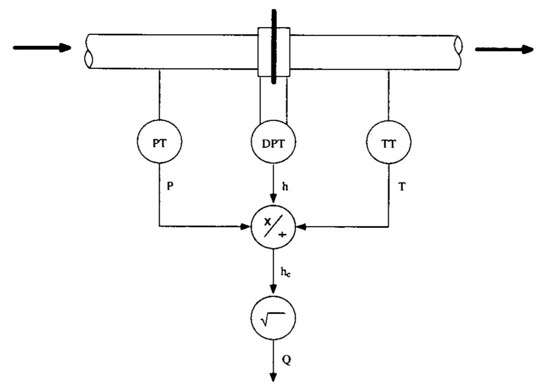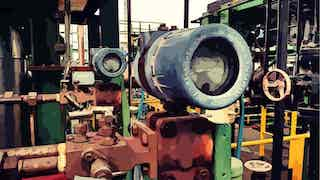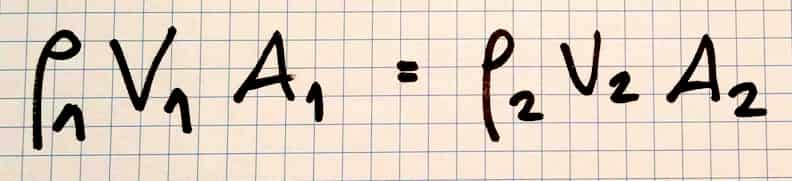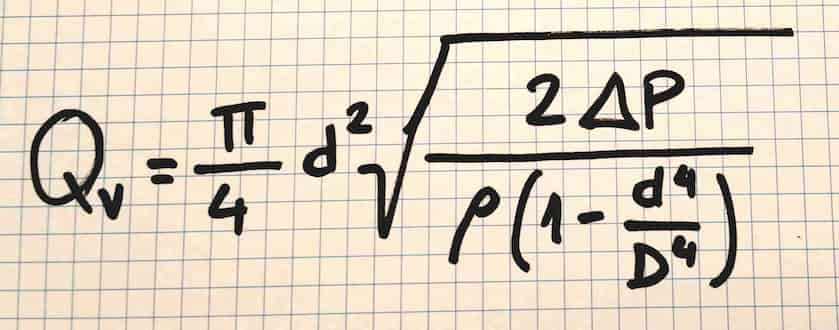# Pressure and Temperature Flow Compensation Formula and equations

Written by Iñaki EseberriHe is a Process Control Lover experienced in complex and high profitable automation systems in the chemical and plastics industry. For a few years now, he has been an Associate Professor at the University' Fluid Mechanics Department and Director of a Postgraduate Degree in Industrial Control.

• Rating: 4.3 - 8 reviews

## What is pressure compensation? and why temperature compensation is required?

This article allows us to understand what is the relationship between the flow measurement, the pressure and the temperature of a fluid. This article shows how the flow measurement is compensated when we have a flow measurement that does not automatically compensate for differences in fluid pressure and temperature. If you are interested in making a hole plate calculation you can visit our calculator pages to make the calculation and download the result in a spreadsheet.

Contents

## 1.What is Flow Compensation?

### Why do you do process temperature and pressure flow compensation?

In steam or gas flow measurement, the density of the steam or gas changes as pressure and temperature change.  This change in density can affect the accuracy of the measured flow rate if it is uncompensated. Temperature, pressure compensated flow control is a flow modified mathematically by one or more additional variables, like :

• Temperature
• Pressure
• Density
• Viscosity

Producing a resultant value for flow compensation that is more accurate. It is compensated for the error effects of the other variables.

### Formula for pressure

This post is related with how to compesate flow with pressure and temperature. If you are looking for the Pressure Formula click here!

Why do you do process temperature and pressure flow compensation?

### Pressure Temperature Compensation Flow Measurement Formula

Orifice meters require Pressure Temperature Compensation Formula when we use them to measure steam or gas flow in pipes with variable operating pressure and temperature. Normally we do not have an online density measurement. In this case we will consider the density constant to simplify the calculations. If you want to perform a density compensation in the flow measurement you must take into account the density variation.DP Orifice Plate Diagram with Pressure and Temperature Transmitters for Flow Compensation

The variations in pressure and temperature have a significant effect on steam or gas density, this is why without this pressure and temperature compensation the flow measurement can have large errors.

This post will help you to understand the basics concepts around this formulas (flow compensation formula) and will aslo let you implement them on your process control system.Differential Pressure Flowmeter based on Orifice Plate Technology installed on process plant

We have prepared this pdf file because sometimes you need this information to discuss with colleagues or simply to implement it in your control systems.

We consider that it is better to have a well-formatted text that includes all the key concepts explained in this publication ready to share or save for later use.

## 2.Obtaining the Real Density Formula

Staring with the ideal gas law, we can extract density from the formula and calculate de density for two different situations (design and real). We need to apply a density compensation in flow measurement.

where :

• P = Pressure
• T = Temperature
• V = Volume
• Mw = Molecular Weight
• n = Number of mols
• R = Gas constant
• rho = steam or gas density

After simplify the previous equation we can have different results depending on the different situations, so we can have a set of design conditions and also a set of real situation conditions....

Operating with the formulas for real density formula and design density we can obtain the formula to define the real density taking in account the pressure and temperature compensation.

## 3.Deriving the Differential Pressure Flow Equation

To obtain the Differential Pressure Flow equation we will use two basic fluid mechanics equations : Eulers equation of continuity and Bernoullis principle.The Eulers Equation for relate velocity and area for a fluid

Where:

• V = linear velocity of fluid
• rho = steam or gas density
• A = Area

And:

Where:

• P = Pressure
• rho = steam or gas density
• V = linear velocity of fluid
• g = standard gravity
• h = height

Rearranging the previous equation and assuming constant density and h1=h2 we have ....

Now its time to express fluids velocity in function of pipe diameter (D) and orifice (d) plate restriction diameter:

and after that, we can substitute V1 inside the previous equation, the resulting equation gives us the velocity at the restriction:

To calculate the volumetric flow we have to multiply both sides by the area of the restriction:Volumetric Flow Depends on Differential Pressure, Density of Gas and Ratio of diameters

If we concentrate all the constants in just constant called K we have the following expressions refering to QV and QM :

## 4.Pressure Temperature Compensation Formula (steam or gas flow compensation formula)

### How does pressure compensated flow control work?

Now its time to substitute the real density by the density calculated in point 2.

After the substitution we will have the following formulas which are used to calculate the compensated flow for every orifice plate flowmeter (depending if you need volumen or mass flow).

Remeber that you must use the flow square root extraction formula in your DCS!!!

## 5. References

• EMERSON (2015) Theory of dP Flow
• MOORE Products (1994) Pressure and temperature compensations of an orifice meter using the model 352 single loop controller
• Wikipedia.org
• EMERSON Rosemount Differential Pressure Flow Gas Flow Measurement
• ISA Maintenance of Instruments & Systems, Second Edition Lawrence D. Goettsche

## Any question?

If you have any question regarding this article, please don't hesitate to get in touch ... Anyway you can have a look at our frequently asked questions page, there you will find a solution to the most common questions.

x